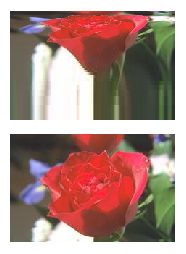# Images with Log-Linear Aspect Ratios

QUESTION: I wish to display an image with a logarithmic aspect ratio on one axis, and a linear aspect ratio on another axis. This is different from displaying the logarithm of the image values, because what I want is that the image pixels at the bottom on the image be stretched more than the pixels at the top.

I can think of some (tortured) ways to do this, but I keep thinking there must be some sophisticated way to handle this in IDL. Do you have any ideas?ANSWER: I don't have any ideas, but Ben Tupper does. He suggests that the way to do this would be to use a flat, filled IDLgrSurface object that is projected in the same way one might view an image, but with a log-scaled Y axis. The image data is placed on top of this surface by means of a texture map, using the TEXTURE_MAP keyword of the surface object. He offers the following IDL code as an example.

```   ; Read the data, collect size information.
rose = Read_Image(FilePath('rose.jpg',SUBDIRECTORY=['examples','data']))
dim = Size(rose, /DIMENSIONS)
nx = dim
ny = dim
x = Findgen(nx) + 1
y = Findgen(ny) + 1
s = Replicate(1, nx, ny)

; The first surface is a "regular" surface.
surf_1 = Obj_New("IDLgrSurface", s, x, y, \$
COLOR=[255,255,255], STYLE=2, \$
TEXTURE_MAP=Obj_New("IDLgrImage", rose))
model_1 = Obj_New("IDLgrModel")
model_1 -> Add, surf_1
model_1 -> Translate, 0, -ny/2. - 10, 0

; Scale so they take up the same amount of space on display.
scale = ny / alog10(ny)

; The second surface is log scale in y.
surf_2 = Obj_New("IDLgrSurface", s, x, alog10(y), \$
COLOR=[255,255,255], STYLE=2, \$
TEXTURE_MAP=Obj_New("IDLgrImage", rose))
model_2 = Obj_New("IDLgrModel")
model_2 -> Add, surf_2
model_2 -> Scale, 1, scale, 1
model_2 -> Translate, 0,ny/2. + 10, 0

; View the two surfaces
XObjView, [model_1, model_2] , /BLOCK

; Clean up.
Obj_Destroy, [model_1, model_2]
```

Running the code above results in the output shown in the figure below. The image with a linear aspect ratio is shown at the bottom of the figure, and the image with a logarithmic Y axis is shown at the top of the figure.The image with logarithmic aspect ratio is at the top.Version of IDL used to prepare this article: IDL 7.0.1.Web Coyote's Guide to IDL Programming